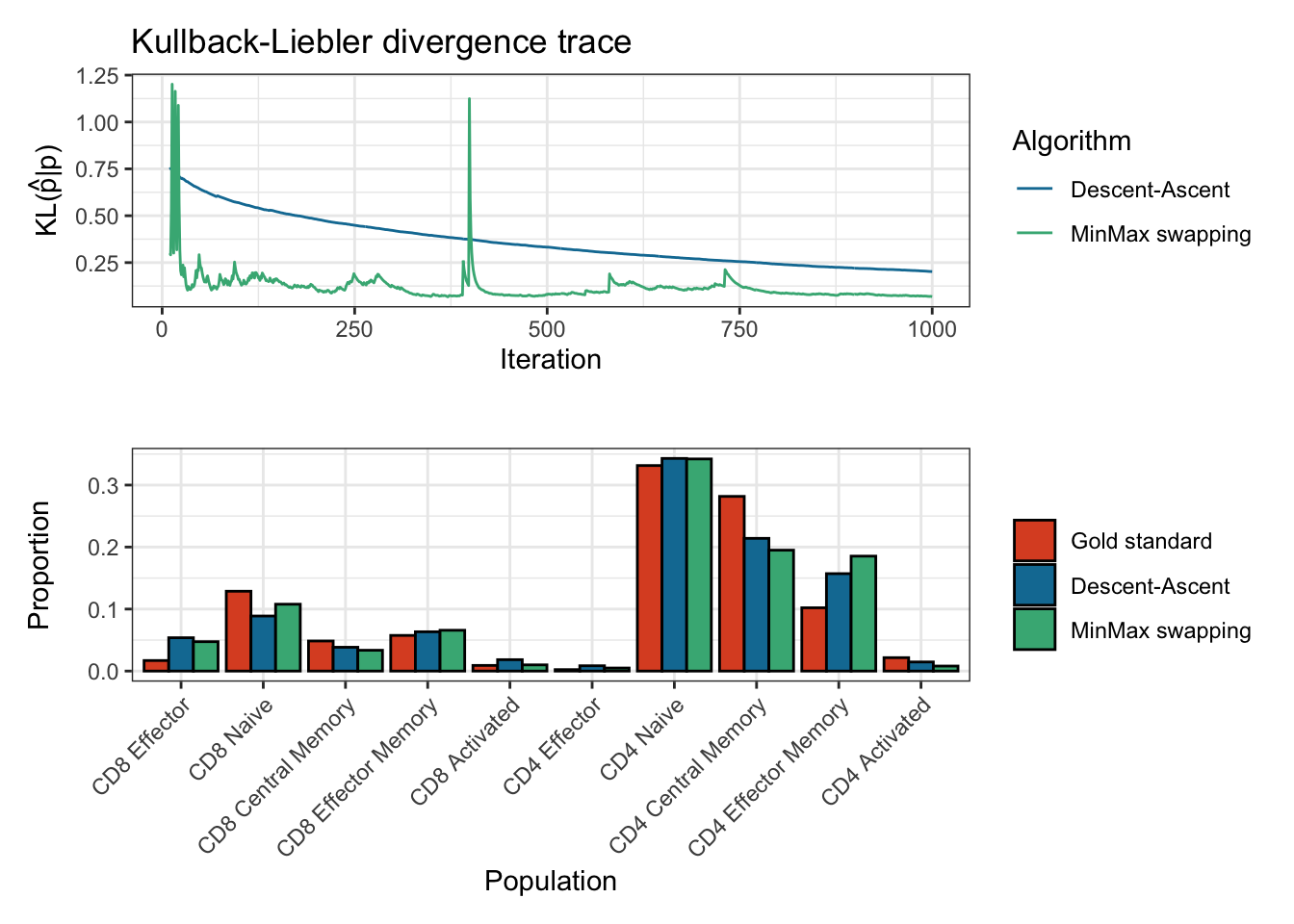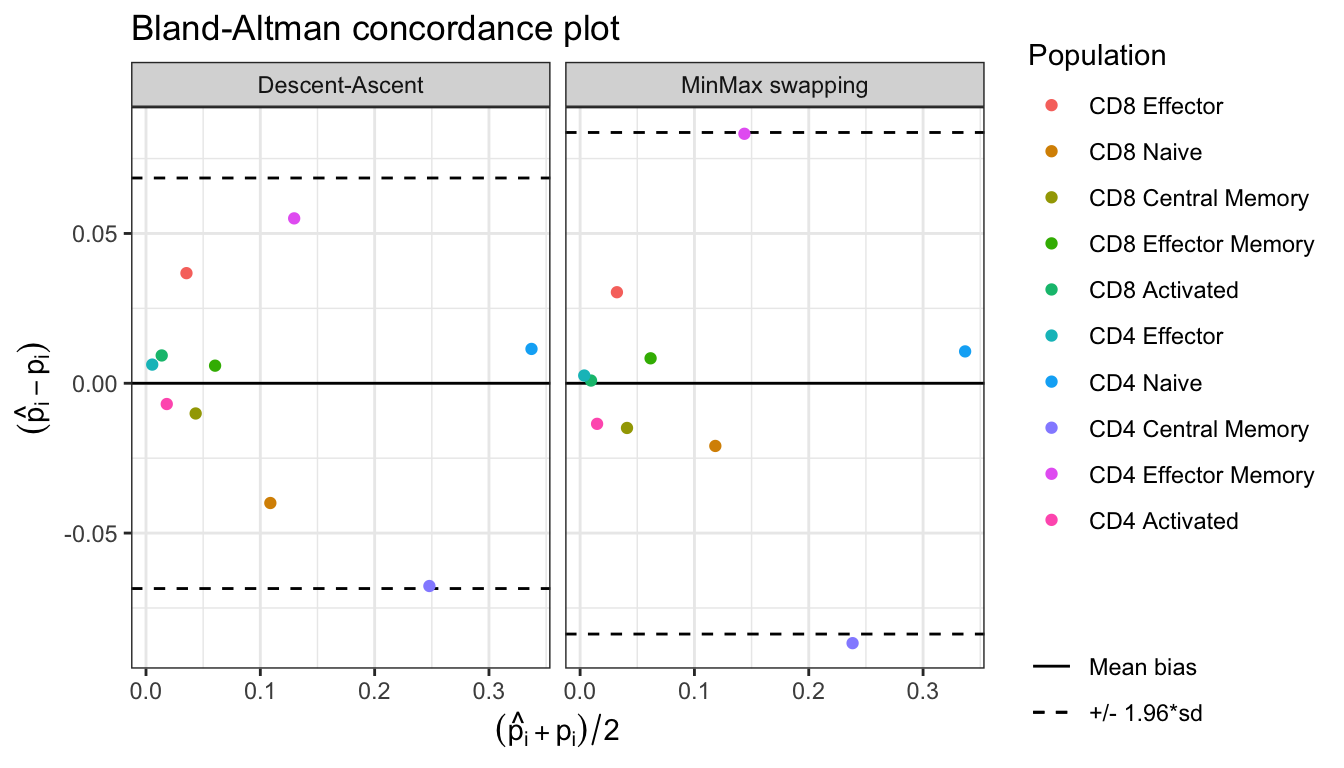# `CytOpT``CytOpT` uses regularized optimal transport to directly estimate the different cell population proportions from a biological sample characterized with flow cytometry measurements.

## Overview

`CytOpT` is an `R` package that provides a new algorithm relying regularized optimal transport to directly estimate the different cell population proportions from a biological sample characterized with flow cytometry measurements. Algorithm is based on the regularized Wasserstein metric to compare cytometry measurements from different samples, thus accounting for possible mis-alignment of a given cell population across sample (due to technical variability from the technology of measurements).

The main function of the package is `CytOpT()`.

The methods implemented in this package are detailed in the following article:

Paul Freulon, Jérémie Bigot, Boris P. Hejblum. CytOpT: Optimal Transport with Domain Adaptation for Interpreting Flow Cytometry data https://arxiv.org/abs/2006.09003

## Installation

You can install and load `CytOpT` into `R` from `CRAN` with the following commands:

``````install.packages("CytOpT")
library(CytOpT)``````

Alternatively, you can install the development version of CytOpT like so:

``````remotes::install_github("sistm/CytOpT-R")
library(CytOpT)``````

## Example

This is a basic example of `CytOpt` usage:

### Data import

``````library(CytOpT)
data("HIPC_Stanford")``````
``````# Define the true proportions in the target data set
gold_standard_manual_prop <- c(table(HIPC_Stanford_1369_1A_labels)/length(HIPC_Stanford_1369_1A_labels))``````

### Proportion estimations using optimal transport and minmax swapping procedures

``````# Run CytOpt and compare the two optimization methods
res <- CytOpT(X_s = HIPC_Stanford_1228_1A, X_t = HIPC_Stanford_1369_1A,
Lab_source = HIPC_Stanford_1228_1A_labels,
theta_true = gold_standard_manual_prop,
eps = 0.0001, lbd = 0.0001, n_iter = 10000, n_stoc=10,
step_grad = 10, step = 5, power = 0.99,
method='both', monitoring=TRUE)
#> Running Descent-ascent optimization...
#> Done in 1.1 mins
#> Running MinMax optimization...
#> Done in 15.3 secs``````
``````summary(res)
#> Estimation of cell proportions with Descent-Ascent and MinMax swapping from CytOpt:
#>                     Gold_standard Descent_ascent      MinMax
#> CD8 Effector          0.017004001    0.051811765 0.044949922
#> CD8 Naive             0.128736173    0.088553804 0.101069760
#> CD8 Central Memory    0.048481996    0.036842527 0.036233461
#> CD8 Effector Memory   0.057484114    0.062380003 0.070825666
#> CD8 Activated         0.009090374    0.017439071 0.005579535
#> CD4 Effector          0.002324076    0.007844185 0.007394887
#> CD4 Naive             0.331460344    0.360283016 0.332733654
#> CD4 Central Memory    0.281713344    0.203949350 0.204808587
#> CD4 Effector Memory   0.102082843    0.156117274 0.169102276
#> CD4 Activated         0.021622735    0.014779005 0.027302251
#>
#> Final Kullback-Leibler divergences:
#>  Descent-Ascent MinMax swapping
#>      0.06534157      0.05700472
#> Number of iterations:
#>  Descent-Ascent MinMax swapping
#>            5000           10000``````
``````plot(res)
#> Plotting KL divergence for iterations 10 to 1000 while there were at least 5000 iterations performed for each method.````````Bland_Altman(res\$proportions)``